# The SPP Procedure

#### Statistics Based on Second-Order Characteristics

Statistics that are based on second-order characteristics include Ripley’s K function, Besag’s L function, and the pair correlation function (also called the g function). To understand why these functions are based on second-order characteristics, see  Illian et al. (2008, p. 223-243). These functions usually involve computation of pairwise distances between points.

The K function of a stationary point process is defined such that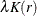is the expected number of points within a distance of r from an arbitrary point of the process. The empirical K function of a set of points is the weighted and renormalized empirical distribution function of the set of pairwise distances between points. The empirical K function can be written as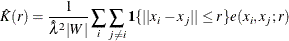where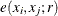is the border edge correction that is described in the section Border Edge Correction for Distance Functions.

For a homogeneous Poisson process,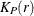can be written as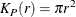Exploratory analysis usually involves computing both the empirical K function,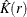, and the K function for a Poisson process,. A comparison of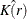andmight indicate clustering or regularity depending on whether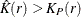or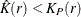.

Besag’s L function is a transformation of the K function and is defined as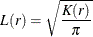For a homogeneous Poisson process,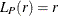.

The pair correlation function, g(r), can also be expressed as a transformation of the K function: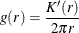Illian et al. (2008), Stoyan (1987), and Fiksel (1988) suggest an alternative expression for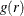: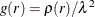where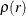is the second-order product density function. Cressie and Collins (2001) provides an expression foras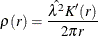where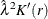can be written as a kernel estimate,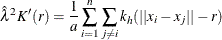where a is the area,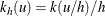, and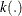is a kernel such as the uniform kernel or the Epanechnikov kernel (Silverman 1986). PROC SPP uses the version that is based on the uniform kernel; for more information about the uniform kernel, see the section Nonparametric Intensity Estimation. Based on the formula for the second-order product densityin terms of the kernel estimate, Stoyan (1987) gives an edge-corrected kernel estimate foras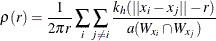Dividingby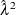gives the pair correlation functionas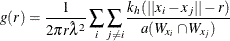where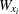indicates the translation of the study area window W by the distance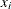from its origin. The above expression forwas given by Stoyan and Stoyan (1994) using the translation edge correction.

A border-edge-corrected version ofcan be written as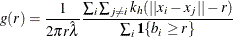whereand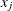are points within the boundary at a distance greater than or equal to r; where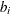is the distance ofto the boundary of W,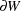; and where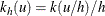for a kernel, such as the uniform kernel or the Epanechnikov kernel. For more information about the uniform kernel, see the section Nonparametric Intensity Estimation. For a homogeneous Poisson process,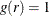. For any point pattern, values ofgreater than 1 indicate clustering or attraction at distance r, whereas values ofless than 1 indicate regularity.